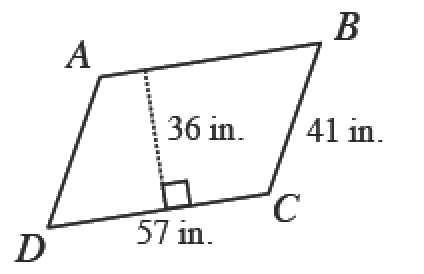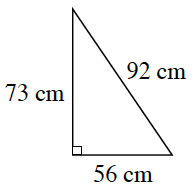### Home > CC2 > Chapter 1 > Lesson 1.2.7 > Problem1-129

1-129.

Find the area and perimeter of each figure below.

a.$ABCD$ is a parallelogram.

The formula to find the area of a parallelogram is (base)(height).

In a parallelogram, opposite sides will have equal length.

$\text{Perimeter}=57\ \text{in.}+41\ \text{in.}+57\ \text{in.}+41\ \text{in.}$
$\text{Perimeter}=196$ in.

$\text{Area}=(36\text{ in.})(57\text{ in.})=2052$ in.2

b.The formula to find the area of a triangle is $\frac{1}{2}\cdot(\text{base})\cdot(\text{height})$

Since this is a right triangle, you can use one of the legs for the height and the other for the base.
Find the perimeter by finding the sum of the given side lengths.